Certificate

### Learn on Scaler Topics and get certified.# How to Reverse a String in Python?

Learn about How to Reverse a String in Python?

There are a plethora of built-in functions present in the python interpreter that are easily available to solve any problem with the most optimal solutions. However, when we take a look at the functions of the string library in python for string manipulation, there does not exist any “reverse()” function hence other methods listed and explained below would be helpful to solve the problem.

## What is a string?

A string by definition is an immutable data type which can’t be changed once declared in a program. Any set of characters enclosed in single or double quotes denote a string.

``````name = "interview"
``````

## Indexing in strings in python:

We use indices to allow for easy access of any character/value in the string. An index is a location for a specific character in a string.

Here is an example of indexing a string.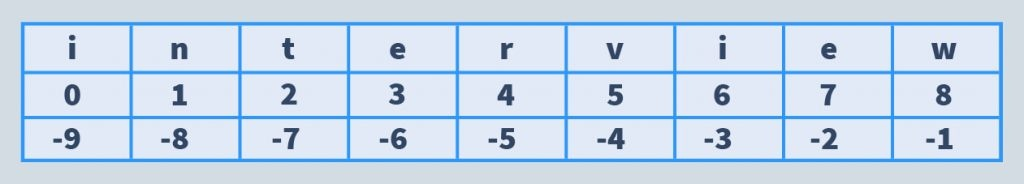To access any character from the string, we can use any of the two values mapped to it, listed in the table above. Given below, is an example for the same.

``````print(name)
print(name[-7])
``````

Output:

``````t
t
``````

## How to reverse a string?

In this article we will discuss reversing a string using the following methods:

1. Using a for loop
2. Using a while loop
3. Using extended slice syntax
4. Using join function
5. Using reversed() function
6. Using recursion
7. Using stack

### Using a For Loop:

In this method, we call a function that reverses a string by creating a new variable (empty string) which will hold our reversed string after we iterate through our input string by adding each character to the beginning of the string.

This method is generally slow because of the loop, as well as the copy we create each time we add a character to the string.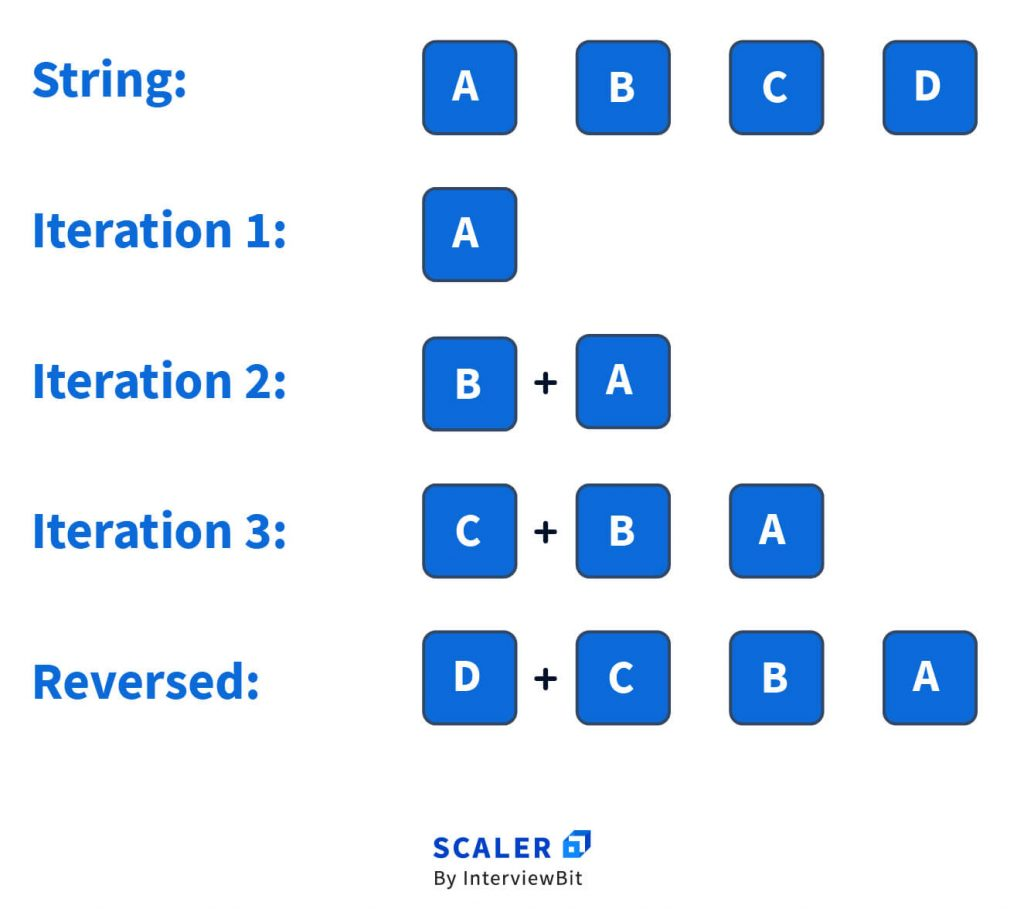Code:

``````def reverse(s: str):
reversed = ""
for ch in s:
reversed = ch + reversed
return reversed

s = "abcd"
print(reverse(s))
``````

Output:

``````dbca
``````

Here’s how the code works:

Initially, the “reversed” is an empty string. We then progress with the for loop that runs for every character (ch) in our string (s).

In the string s, the for loop starts with the character ‘a’. Our statement

reversed = ch + reversed

makes the current reversed string = a. In the next iteration of the for loop, the character is ‘b’, and as per the statement, it becomes

b + the current reversed string which is just ‘a’, hence making the reversed string = ba.

This continues till the last character, reversing the string.

### Using a While Loop:

To reverse a string using a while loop in python, we require a pointer that points to the last character in the string. The built-in “len()” function, that returns the length of a string, can be used to set the initial value of the pointer.

As we keep decrementing the value of the pointer, it points to the characters that are required in reversing the string. The time complexity of this approach is O(n).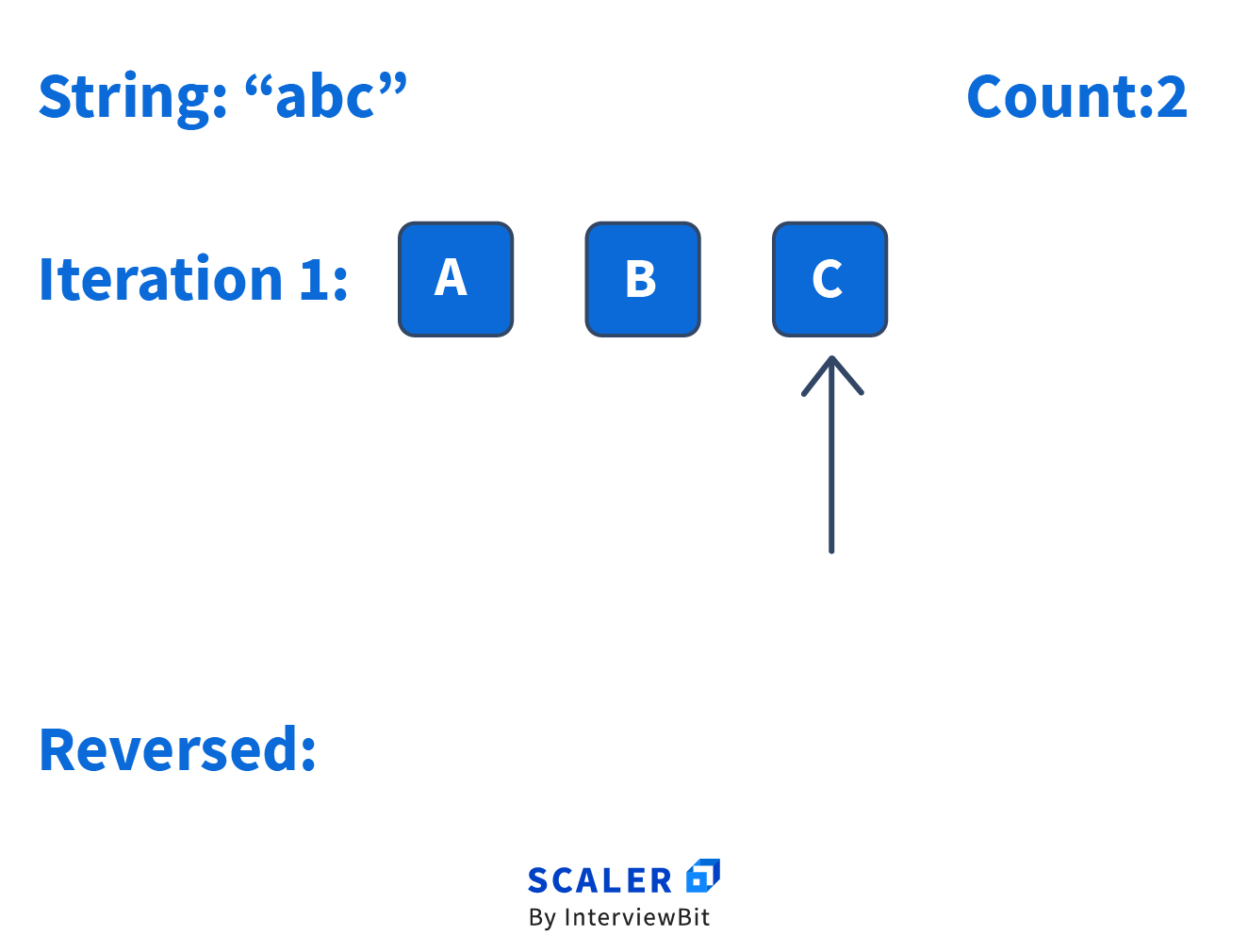Code:

``````def reverse(s):
reversed = ""
count = len(s)
while count > 0:
reversed += s[count - 1]
count = count - 1
return reversed

s = "interview"
print(reverse(s))
``````

Output:

``````weivretni
``````

### Using Extended Slice Syntax:

A simple way to understand the extended slice syntax is slice[start:stop]. The slice starts at the location defined by start, stops before the location stop is reached, and moves starting with one position then onto the next by step items.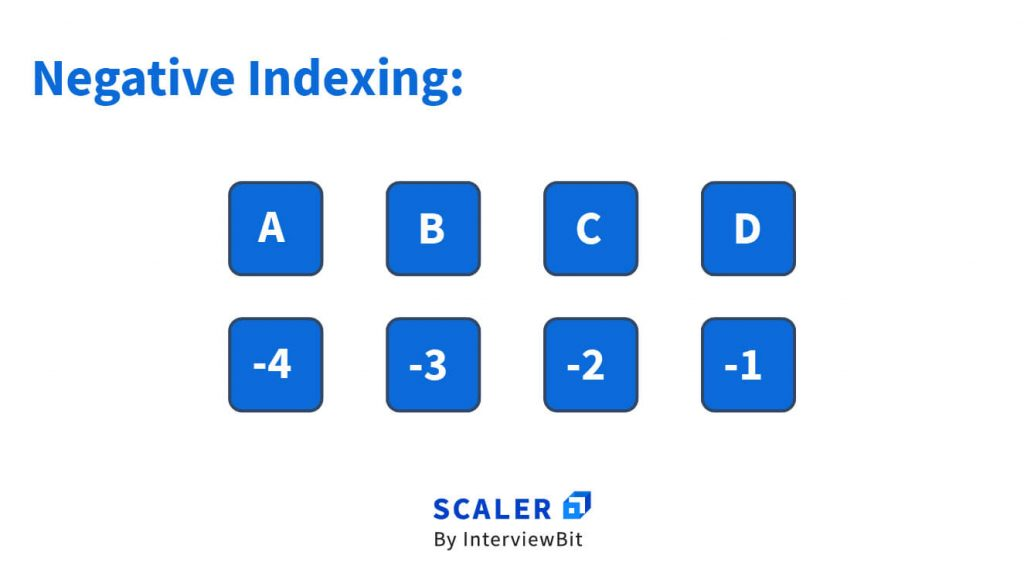[-1:0:-1] would mean that we start from the last index len(string)-1 going up to 0 which isn’t included, taking a step of -1 which here means, reverse.

Say we slice the string like – string[::2]. This essentially represents string[0:-1:2], which means moving from the start, till the end, with steps of 2.

Hence the use of string[::-1] would reverse the string, as we would be moving in negative steps.

The extended slice syntax comes under list comprehension and is the fastest method to reverse a string.

Code:

``````def reverse(s):
return s[::-1]
s = "interview"
print(reverse(s))
``````

Output:

``````weivretni
``````

### Using join() and reversed() Function:

Python provides a function called “reversed()“ for lists, which returns a special reversed iterator object. An iterator object is essentially something that needs to be iterated over (or looped over) to produce its elements.

If we do not give it to .join it would just print out repr of the reversed object: <reversed object at 0x7f2ba03be321>.

A repr is a string representation of an object that can be evaluated, it is essentially something that could be used to recreate the object.

eg :

``````>>> from decimal import Decimal
>>> a = Decimal(1.25)
>>> print(a) 1.25 <---- this is from __str__
>>> a Decimal('1.25') <---- this is from __repr__
``````

We give “”.join an iterable as an argument, which here is the reversed object and joins the elements to produce the string.

There are other approaches to consume it without join that bring about a different object. For instance, list takes the reversed object and creates a list out of the elements it produces:

``````a = reversed('pqrs')
print(list(a))
``````

Output:

``````['s', 'r', 'q', 'p']
``````

If you need a string in the end, then the best option is to use .join.

Code:

``````def reverse(s):
reversed_str = "".join(reversed(s))
return reversed_str
``````

### Using recursion:

We can reverse a string using recursion by finding what we call – base case and recursive cases. A small observation that we can make here is that if the length of the string is equal to 0, the string can be returned, which will be the base case.

The recursive case would be when the length of the string is not equal to 0. In this case, we call the reverse function recursively and slice the string in such a way that we only leave the first character, later on concatenating it to the end of the sliced string.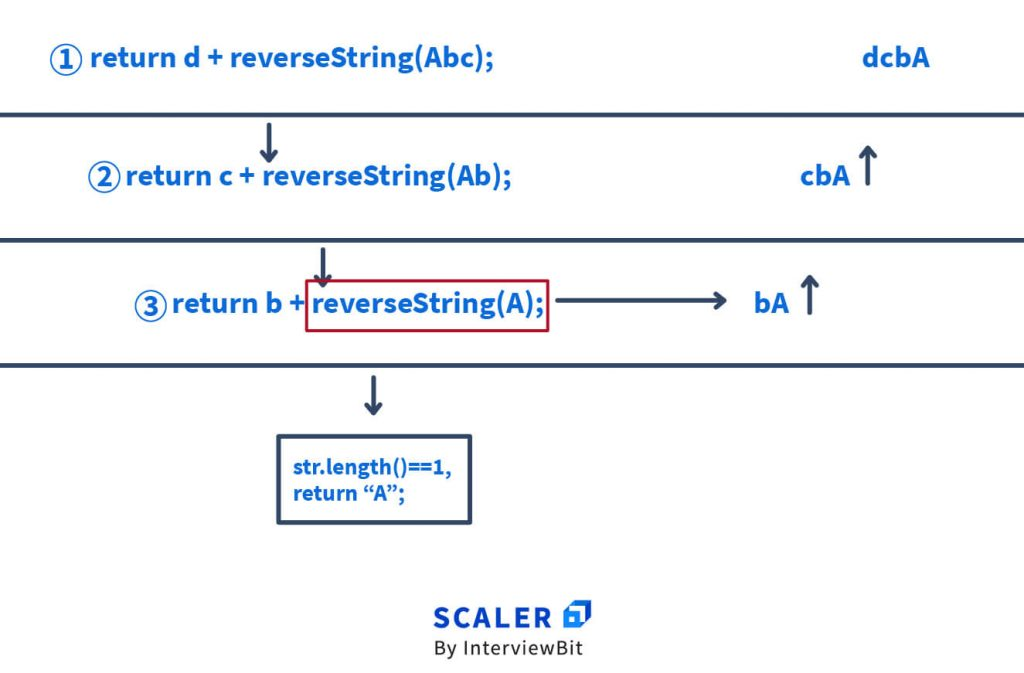Code:

``````def reverse(s):
if len(s) == 0:  # base case
return s
else:
# adding the first character at the end after slicing
return reverse(s[1:]) + s

s = "interview"
print(reverse(s))
``````

Output:

``````weivretni
``````

### Using stack:

Stack is a “Last In First Out(LIFO)” type of data structure. This means that the last character that would be pushed into the stack will be the first to be printed out. This helps in reversing the string. One by one each character will be pushed into the stack and then popped out, returning the reversed string.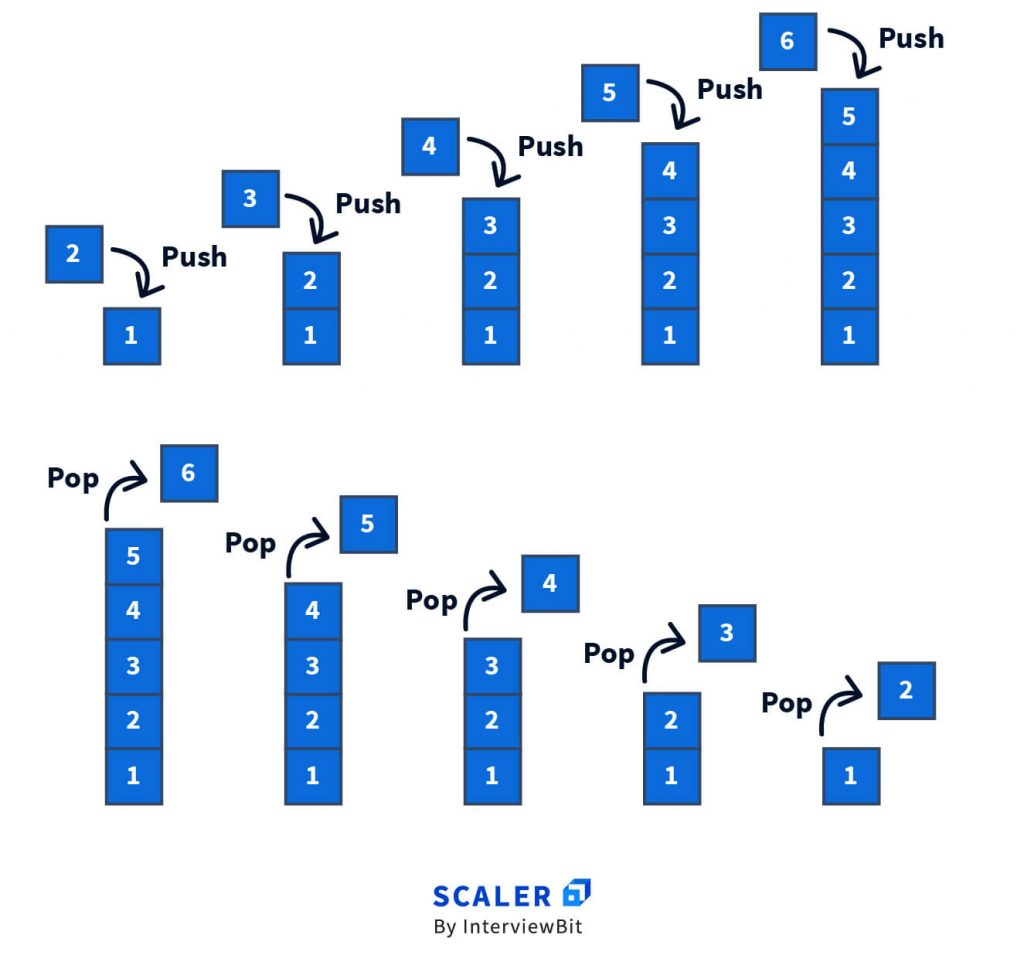Code:

``````def reverse(s: str):
stack = []
reversed_string = ""
for ch in s:
stack.append(ch)
while len(stack):
reversed_string += stack.pop()
return reversed_string

print(reverse("abc"))
``````

Output:

``````cba
``````

## Conclusion:

In the above methods we have successfully reversed a string using python. Given below is a short gist of the approaches used:

• In python, strings are immutable. When a string is changed, it isn’t modified, a new string is created
• Strings are sliceable. When you slice a string, it gives you a new string from one point in the string, forwards or backwards, to another point, by given “steps” or increments. It is also the most performant approach
• The method used for reversing a string using slicing is – string[::-1]
• Another method of reversing a string is to use built-in functions of python which are although more readable, but also about 3 times slower
• ”.join(reversed(string))
• A notion to keep in mind is that strings are iterables, hence a string can also be reversed by starting first with an empty string and building up a new one using a while loop with pure syntax and literals or by using a for loop
• A method that doesn’t use O(n) space, and doesn’t create a new string is the two pointer approach, wherein we swap the first and last characters, moving towards the middle of the string.